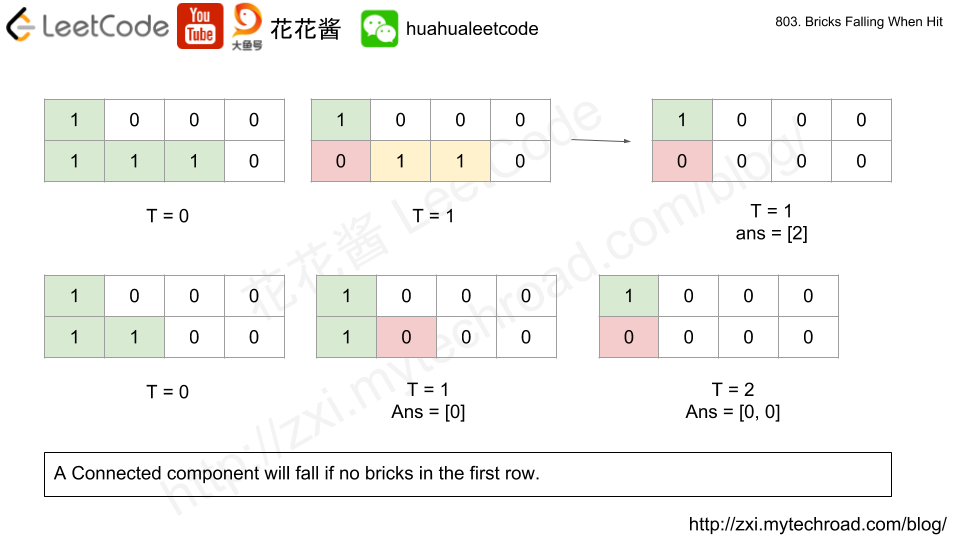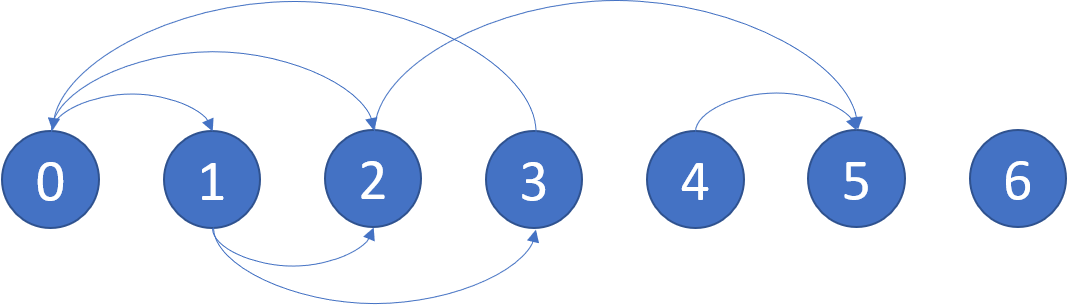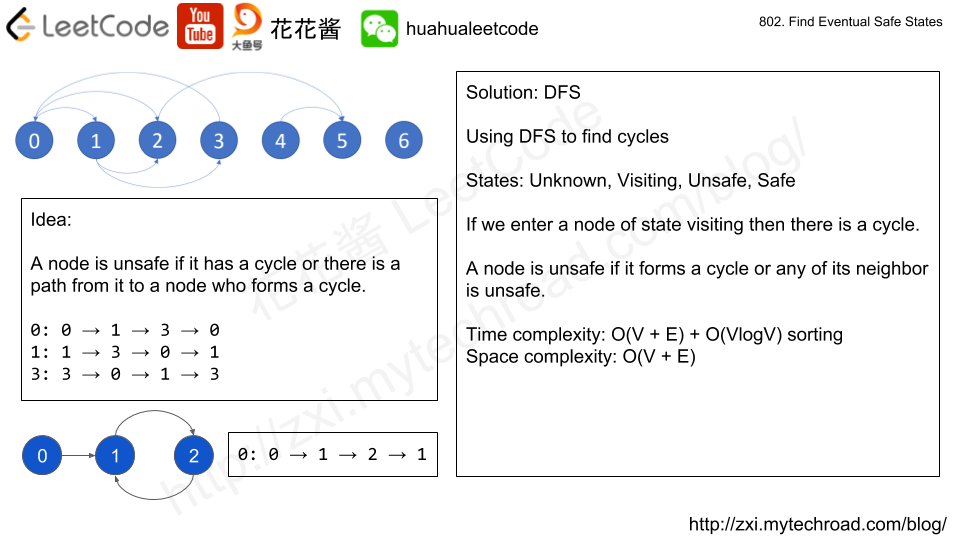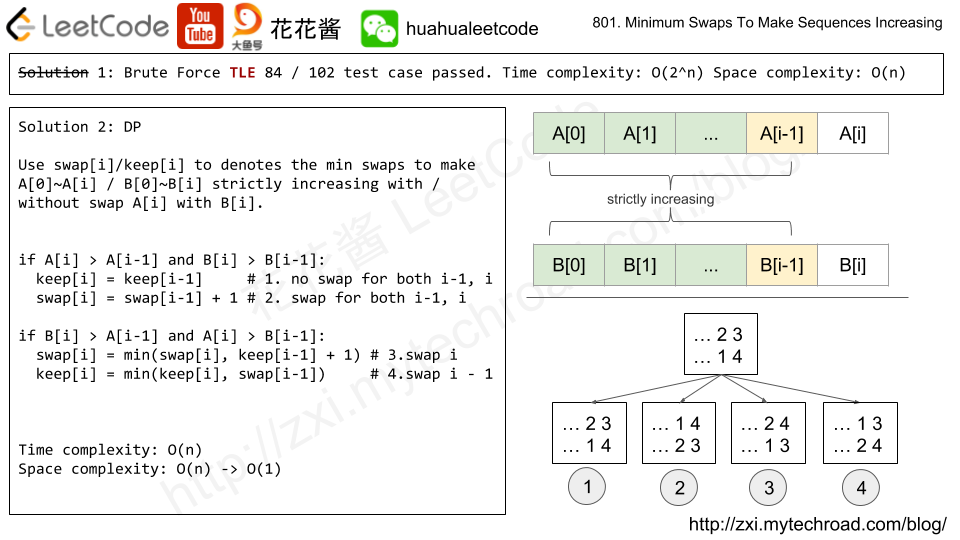# Problem

We have a grid of 1s and 0s; the 1s in a cell represent bricks.  A brick will not drop if and only if it is directly connected to the top of the grid, or at least one of its (4-way) adjacent bricks will not drop.

We will do some erasures sequentially. Each time we want to do the erasure at the location (i, j), the brick (if it exists) on that location will disappear, and then some other bricks may drop because of that erasure.

Return an array representing the number of bricks that will drop after each erasure in sequence.

Example 1:
Input:
grid = [[1,0,0,0],[1,1,1,0]]
hits = [[1,0]]
Output: 
Explanation:
If we erase the brick at (1, 0), the brick at (1, 1) and (1, 2) will drop. So we should return 2.
Example 2:
Input:
grid = [[1,0,0,0],[1,1,0,0]]
hits = [[1,1],[1,0]]
Output: [0,0]
Explanation:
When we erase the brick at (1, 0), the brick at (1, 1) has already disappeared due to the last move. So each erasure will cause no bricks dropping.  Note that the erased brick (1, 0) will not be counted as a dropped brick.

# Idea

1. For each day, hit and clear the specified brick.
2. Find all connected components (CCs) using DFS.
3. For each CC, if there is no brick that is on the first row that the entire cc will drop. Clear those CCs.# Problem

https://leetcode.com/problems/find-eventual-safe-states/description/

In a directed graph, we start at some node and every turn, walk along a directed edge of the graph.  If we reach a node that is terminal (that is, it has no outgoing directed edges), we stop.

Now, say our starting node is eventually safe if and only if we must eventually walk to a terminal node.  More specifically, there exists a natural number K so that for any choice of where to walk, we must have stopped at a terminal node in less than K steps.

Which nodes are eventually safe?  Return them as an array in sorted order.

The directed graph has N nodes with labels 0, 1, ..., N-1, where N is the length of graph.  The graph is given in the following form: graph[i] is a list of labels j such that (i, j) is a directed edge of the graph.

Example:
Input: graph = [[1,2],[2,3],,,,[],[]]
Output: [2,4,5,6]
Here is a diagram of the above graph.Note:

• graph will have length at most 10000.
• The number of edges in the graph will not exceed 32000.
• Each graph[i] will be a sorted list of different integers, chosen within the range [0, graph.length - 1].

# Idea: Finding Cycles# Solution 1: DFS

A node is safe if and only if: itself and all of its neighbors do not have any cycles.

Time complexity: O(V + E)

Space complexity: O(V + E)

# Problem

https://leetcode.com/problems/minimum-swaps-to-make-sequences-increasing/description/

We have two integer sequences A and B of the same non-zero length.

We are allowed to swap elements A[i] and B[i].  Note that both elements are in the same index position in their respective sequences.

At the end of some number of swaps, A and B are both strictly increasing.  (A sequence is strictly increasing if and only if A < A < A < ... < A[A.length - 1].)

Given A and B, return the minimum number of swaps to make both sequences strictly increasing.  It is guaranteed that the given input always makes it possible.

Example:
Input: A = [1,3,5,4], B = [1,2,3,7]
Output: 1
Explanation:
Swap A and B.  Then the sequences are:
A = [1, 3, 5, 7] and B = [1, 2, 3, 4]
which are both strictly increasing.


Note:

• A, B are arrays with the same length, and that length will be in the range [1, 1000].
• A[i], B[i] are integer values in the range [0, 2000].# Solution: Search/DFS (TLE)

Time complexity: O(2^n)

Space complexity: O(n)

# Solution: DP

Time complexity: O(n)

Space complexity: O(n)

# Problem

In the following, every capital letter represents some hexadecimal digit from 0 to f.

The red-green-blue color "#AABBCC" can be written as "#ABC" in shorthand.  For example, "#15c" is shorthand for the color "#1155cc".

Now, say the similarity between two colors "#ABCDEF" and "#UVWXYZ" is -(AB - UV)^2 - (CD - WX)^2 - (EF - YZ)^2.

Given the color "#ABCDEF", return a 7 character color that is most similar to #ABCDEF, and has a shorthand (that is, it can be represented as some "#XYZ"

Example 1:
Input: color = "#09f166"
Output: "#11ee66"
Explanation:
The similarity is -(0x09 - 0x11)^2 -(0xf1 - 0xee)^2 - (0x66 - 0x66)^2 = -64 -9 -0 = -73.
This is the highest among any shorthand color.


## Note:

• color is a string of length 7.
• color is a valid RGB color: for i > 0color[i] is a hexadecimal digit from 0 to f
• Any answer which has the same (highest) similarity as the best answer will be accepted.
• All inputs and outputs should use lowercase letters, and the output is 7 characters.

# Solution: Brute Force

R, G, B are independent, find the closest color for each channel separately.

Time complexity: O(3 * 16)

Space complexity: O(1)

# Problem

Given two non-negative integers num1 and num2 represented as string, return the sum of num1 and num2.

Note:

1. The length of both num1 and num2 is < 5100.
2. Both num1 and num2 contains only digits 0-9.
3. Both num1 and num2 does not contain any leading zero.
4. You must not use any built-in BigInteger library or convert the inputs to integer directly.

# Solution: Brute Force

Time complexity: O(n)

Space complexity: O(n)

C++

Mission News Theme by Compete Themes.